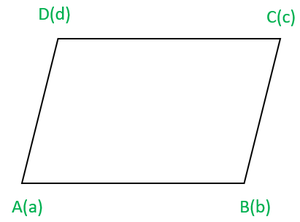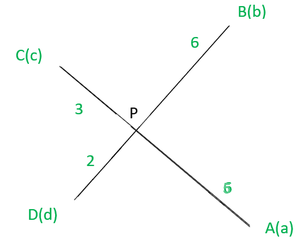Class 12 RD Sharma Solutions – Chapter 23 Algebra of Vectors – Exercise 23.3

• Last Updated : 13 Jan, 2021

Question 1. Find the position vector of a point R which divides the line joining the two points P and Q with position vectorsandrespectively in the ratio 1:2 internally and externally.

Solution:

The point R divides the line joining points P and Q in the ratio 1:2 internally.

The position vector of R =Point R divides the line joining P and Q in the ratio 1:2 externally.

The position vector of R =Question 2. Letandbe the position vectors of the four distinct points A, B, C, D. Ifthen show that ABCD is a parallelogram.Solution:

Given that  are the position vectors of the four distinct points A, B, C, D

such thatGiven that,So, AB is parallel and equal to DC

Hence, ABCD is a parallelogram.

Question 3.Ifare the position vectors of A, B respectively, find the position vector of a point C in AB produced such that AC = 3AB and that a point D in BA produced such that  BD = 2BA.

Solution:

Given thatare the position vector of A and B

Let C be a point in AB produced such that AC = 3AB.

From the given data we can say that point C divides the line AB in

Ratio 3:2 externally. So, the position vector of point C can be written as=D be a point in BA produced such that BD = 2BA

It is clear that point D divides the line in 1:2 externally.

Then the position vectorcan be written as=HenceandQuestion 4.Show that the four points A, B, C, D with position vectorsandrespectively such thatare coplanar. Also, find the position vector of the point of intersection of the lines AC and BD.Solution:

Given thatSum of the coefficients on both sides of the given equation is 8

so, divide the equation by 8 on both the sidesIt is clear that the position vector of a point P dividing Ac in the

Ratio 3:5 is same as that of point P diving BD in the ratio 2:6.

Point P is common to AC and BD. Hence, P is the point of intersection of AC and BD.

Therefore, A, B, C and D are coplanar.

The position vector of point P can be written asorQuestion 5: Show that the four points P, Q, R, S with position vectorsandrespectively such thatare coplanar. Also, find the position vector of the point of intersection of the lines PR and QS.

Solution:

Given thatHereandare the position vectors of point P, Q, R, S-(1)

Sum of the coefficients on both the sides of the equation (1) is 11.

So divide the equation (1) by 11 on both sides.It shows that position vector of a point A dividing PR in the ratio of 6:5 and

QS in the ratio 9:2. So A is the common point to PR and QS.

Therefore, P, Q, R and S are coplanar.

The position vector of point A is given byorQuestion 6:The vertices A, B, C of triangle ABC have respectively position vectorswith respect to a given origin O. Show that the point D where the bisector ofmeets BC has position vectorwhere. Hence deduce that the incentre I has position vectorwhereSolution:

Let ABC be a triangle and the position vectors of A, B, C with respect to some origin say O be

Let D be the point on BC where the bisector ofmeets.be the position vector of D which divides BC internally in the ratioandwhereThus,Therefore, by section formula, the position vector of D is given byLetIncentre is the concurrent point of angle bisectors.

Thus, Incentre divides the line AD in the ratioand

the position vector of incentre is equal toMy Personal Notes arrow_drop_up